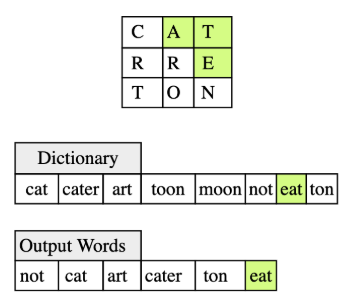# Boggle

## Problem Statement

Given a N×N grid of characters and a dictionary, find all words which can be made from the characters in the grid and are present in the given dictionary.

A word can start and end at any character in the grid. The next character must be adjacent to the previous character in any of the directions: up, down, left, right and diagonal.

The character at each position in the grid can be used only once while making a word.

Suppose we have a 3×3 grid and a dictionary as input. Six words can be made from the grid which are present in the dictionary. Green highlighted characters indicate that they form the word EAT in the grid which is also present in the dictionary.

In the grid, we start at character E, then move to upper diagonal for A and then right to T to make EAT.• Backtracking

## Try it yourself

Your code will be tested for the grid:

grid = [['c', 'a', 't'],
['r', 'r', 'e'],
['t', 'o', 'n']]


and the dictionary:

dictionary = {"cat", "cater", "cartoon", "toon", "moon", "not", "tone", "apple", "ton", "art"}

class Boggle {  public:    Boggle(const vector<vector<char>>& grid, const unordered_set<string>& dictionary){      //TODO: Write - Your - Code    }    unordered_set<string> find_all_words(){      //TODO: Write - your - Code      unordered_set<string> words;       return words;    }};

## Solution

class Boggle {  // code assumes that both dimensions of grid are same  public:    vector<vector<char>> grid;    unordered_set<string> dictionary;    vector<vector<bool>> state;    list<pair<int, int>> find_all_nbrs(int x, int y) {      list<pair<int, int>> nbrs;      int start_x = std::max(0, x - 1);      int start_y = std::max(0, y - 1);      int end_x = std::min((int)(grid.size() - 1), x + 1);      int end_y = std::min((int)(grid.size() - 1), y + 1);      for (int i = start_x; i <= end_x; ++i) {        for (int j = start_y; j <= end_y; ++j) {          if (state[i][j]) {            continue;          }          nbrs.push_back(pair<int, int>(i, j));        }      }      return nbrs;    }    void find_words_rec(int i, int j,string& current,unordered_set<string>& words) {            if (!current.empty() && dictionary.find(current) != dictionary.end()) {        words.insert(current);      }      // we can really speed up our algorithm if we have prefix method available      // for our dictionary by using code like below      /*      if (!dictionary.is_prefix(current)) {        // if current word is not prefix of any word in dictionary        // we don't need to continue with search        return;      }      */      list<pair<int, int>> nbrs = find_all_nbrs(i, j);      for (auto& pr : nbrs) {              current += grid[pr.first][pr.second];        state[pr.first][pr.second] = true;        find_words_rec(pr.first, pr.second, current, words);        current.resize(current.size() - 1);        state[pr.first][pr.second] = false;      }    }    Boggle(const vector<vector<char>>& g, const unordered_set<string>& d) : grid(g), dictionary(d) {       state.resize(g.size());      for (vector<bool>& v : state) {        v.resize(g.back().size());      }    }    unordered_set<string> find_all_words(){      unordered_set<string> words;      string current_word;      for (int i = 0; i < grid.size(); ++i) {        for (int j = 0; j < grid.size(); ++j) {          find_words_rec(i, j, current_word, words);        }      }      return words;    }};int main(){  vector<vector<char>> grid = {{'c', 'a', 't'},                               {'r', 'r', 'e'},                               {'t', 'o', 'n'}};    unordered_set<string> dictionary = {"cat", "cater", "cartoon", "art", "toon", "moon", "eat", "ton"};  Boggle b(grid, dictionary);  unordered_set<string> words = b.find_all_words();  for (auto& s: words) {    cout << s << endl;  }  return 0;}

## Solution Explanation

### Runtime complexity

The runtime complexity of this solution is exponential, $O(N^{N})$, where N is the dimension of the grid.

### Memory complexity

The memory complexity of this solution is quadratic, $O(N^{2})$, where N is the dimension of the grid.

### Solution Breakdown

This is a backtracking problem. We start with one character in the grid and try to make as many words as possible starting with that character. We repeat the same process for all characters in the grid.

To find all the words starting with a character, we use an algorithm very similar to depth-first search. As every character from the grid can appear only once in a word, we’ll need to maintain a boolean matrix to indicate if the corresponding character in the grid is used to make this word.

We can really speed up our algorithm if we have a prefix method available for our dictionary.

Let’s consider an example below where we want to find all words starting with character $E$ (in $2^{nd}$ row and $3^{rd}$ column of the example grid above).

For this example we’ll assume that the dictionary has a prefix() method which returns true if a string is a prefix of a valid word.

• We’ll start with $E$. From our dictionary we know that $E$ is a prefix of a word, so we’ll keep $E$ and continue after marking $E$ as used in the boolean matrix.

• $E$ has $5$ unused neighbors, i.e., $A$, $T$, $R$, $O$, $N$. First we’ll proceed with first neighbor i.e. $A$. $EA$ is a valid prefix.

• $A$ has 4 unused neighbors i.e. $C$, $T$, $R$, $R$. $E$ is a neighbor of $A$, but we’ll not consider it as it is already used. First, we’ll try $C$ but $EAC$ is neither a valid word nor a prefix to valid words in our dictionary. So, we’ll move to the next neighbor, $T$ and $EAT$ is a valid word.

• We’ll add $EAT$ to the output words list and then continue to neighbors of $T$. But no neighbor of $T$ can be part of a prefix or a valid word, so we’ll backtrack and continue with remaining neighbors of $A$ from the previous step.

• In this case, they are $R$, $R$ and $EAR$, $EAR$ is neither a valid word nor prefix a to a valid word in the dictionary, so we’ll backtrack.

• While backtracking we always reset used flags so that this character can be used in some other path. We’ll backtrack once again and will look at other neighbors of $E$, i.e., $T$, $R$, $O$, $N$. We’ll continue until all paths starting from $E$ are explored.

Practice problems like this and many more by checking out our Grokking the Coding Interview: Patterns for Coding Questions course!

Learn in-demand tech skills in half the time